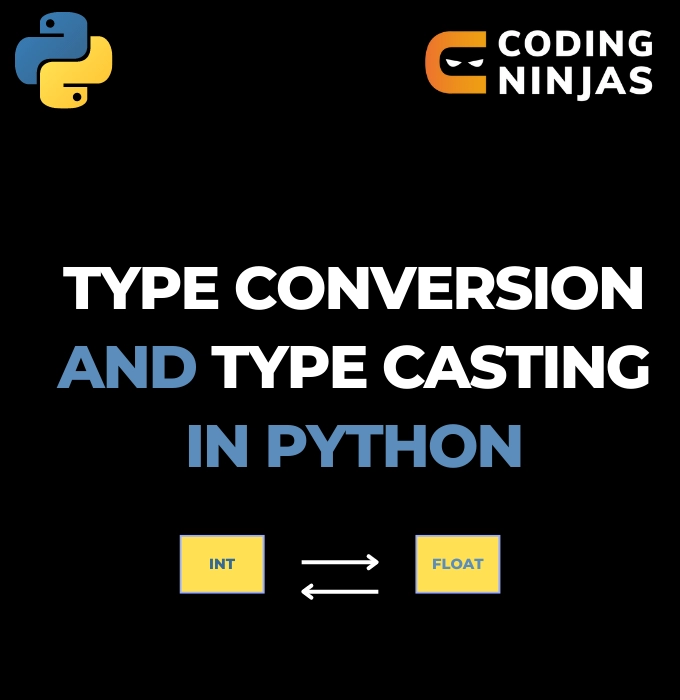New update is available. Click here to update.
Last Updated: Jul 1, 2023
Easy

# Type Conversion & Type Casting in PythonKomal0 upvotes

## Introduction

A significant part of programming involves playing around with data. While performing some tasks, situations might arise where the data type has to be changed from one type to another.

For example, if the variable is of type string, that has to be converted to int in order to perform arithmetic operations. In such a condition, the concept of type conversion and type casting comes into play.Before learning Type Conversion and Type Casting in Python, you should be familiar with Python Data Types. So check out the blog Python Data Types for Beginners to get a better understanding.

## Type Conversion

In type conversion, the Python interpreter automatically converts one data type to another. Since Python handles the implicit data type conversion, the programmer does not have to convert the data type into another type explicitly.

The data type to which the conversion happens is called the destination data type, and the data type from which the conversion happens is called the source data type.

In type conversion, the destination data of a smaller size is converted to the source data type of larger size. This avoids the loss of data and makes the conversion safe to use.

In type conversion, the source data type with a smaller size is converted into the destination data type with a larger size.

Let’s see an example to understand type conversion in Python.

``````intType = 20
floatType = 0.8

# intType is converted from int to float
result = intType + floatType

# intType is of type int
print("datatype of intType:", type(intType))

# floatType is of type float
print("datatype of floatType:", type(floatType))

print("intType + floatType = ", result)
# result is of type float
print("result: ", type(result))``````

Output:

``````datatype of intType: <class 'int'>
datatype of floatType: <class 'float'>
intType + floatType =  20.8
result:  <class 'float'>``````

Let’s now try to add a string of larger size and int of smaller size.

``````intType = 20
strType = "0.8"

# add an integer and string
result = intType + strType

print("Data type of the listType :",type(intType))
print("Data type of the listType :",type(strType))

print("result: "+result)
print("Data type of the listType :",type(result))``````

Output:

``````Traceback (most recent call last):
File "<string>", line 5, in <module>
TypeError: unsupported operand type(s) for +: 'int' and 'str'``````

As you might have guessed, we encountered a TypeError while running the above code. Python does not do the implicit conversion in this case. However, this error can be resolved using type casting, which will be discussed next.

Also See, Divmod in Python, Swapcase in Python

## Type Casting

In type casting, the programmer has to change the data type as per their requirement manually. In this, the programmer explicitly converts the data type using predefined functions like int(), float(), str(), etc. There is a chance of data loss in this case if a particular data type is converted to another data type of a smaller size.

The in-built Python Functions used for the conversion are given below, along with their descriptions:

Now let’s see an example to understand type casting in Python.

``````strType = "CodingNinjas"

# printing string converting to tuple
tupleType = tuple(strType)
print("After converting string to tuple : ")
print(tupleType)
print("Data type of the tupleType :",type(tupleType))

# printing string converting to set
setType = set(strType)
print("After converting string to set : ")
print(setType)
print("Data type of the setType :",type(setType))

# printing string converting to list
listType = list(strType)
print("After converting string to list : ")
print(listType)
print("Data type of the listType :",type(listType))

``````

Output:

``````After converting string to tuple :
('C', 'o', 'd', 'i', 'n', 'g', 'N', 'i', 'n', 'j', 'a', 's')
Data type of the tupleType : <class 'tuple'>
After converting string to set :
{'g', 'N', 'n', 'C', 's', 'o', 'j', 'd', 'a', 'i'}
Data type of the setType : <class 'set'>
After converting string to list :
['C', 'o', 'd', 'i', 'n', 'g', 'N', 'i', 'n', 'j', 'a', 's']
Data type of the listType : <class 'list'>``````

Must Recommended Topic, Floor Division in Python and Convert String to List Python.

Check out Escape Sequence in Python

### What is meant by type conversion and type casting in Python?

In type conversion, the Python interpreter automatically converts one data type to another. In type casting, the programmer converts the data type as per their requirement manually.

### What are the common methods used to change data types in Python?

Type Conversion and Type Casting in Python enable converting one data type into another.

### Differentiate between type conversion and type casting in Python based on data loss.

There is a chance of data loss in type casting while the data is safe in type conversion.

### How does data conversion take place in type casting in Python?

In explicit type casting, the data types are converted manually by the programmer using in-built functions.

### Is it possible to convert a string to an integer in Python?

No, it is not possible to convert a string to an integer even by using the in-built int() method.

## Conclusion

That was all about Type Conversion and Type Casting in Python. This blog covered various concepts, examples, and frequently asked questions relating to this topic were covered in detail. Now that you know what Type Conversion and Type Casting are, go ahead and attempt some questions on our Coding Ninjas Studio Platform!